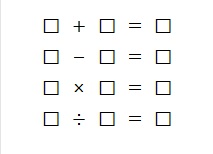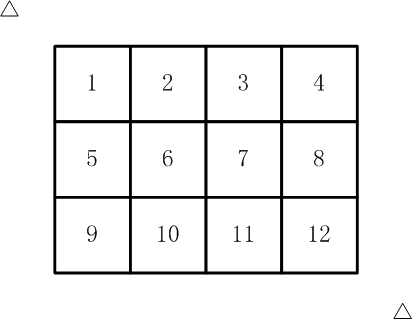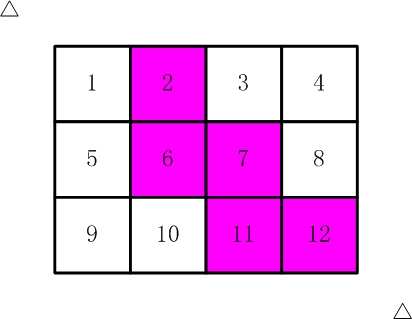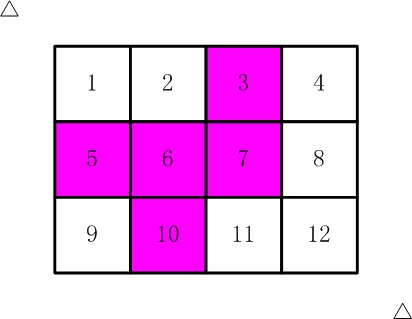## 2016年第七届蓝桥杯省赛试题及详解（Java本科A组）

### 蓝桥杯历年真题题目及题解目录汇总

1. 结果填空 (满分3分)
2. 结果填空 (满分5分)
3. 结果填空 (满分9分)
4. 代码填空 (满分11分)
5. 代码填空 (满分13分)
6. 结果填空 (满分15分)
7. 结果填空 (满分19分)
8. 程序设计（满分21分）
9. 程序设计（满分23分）
10. 程序设计（满分31分）

1.煤球数目

....

``````public class _01煤球数目 {

public static void main(String[] args) {
int ans=0,s=0;
for(int i=1;i<=100;i++) {
s+=i;
ans+=s;
}
System.out.println(ans+" "+s);
}

}``````

2.生日蜡烛

``````public class _02生日蜡烛 {

public static void main(String[] args) {

for(int i=1;i<=150;i++) {
int s=0;
for(int j=i;j<=150;j++) {
s+=j;
if(s==236)
System.out.println(i);
if(s>236)
break;
}
}

}

}``````

3.搭积木

0
1 2
3 4 5
6 7 8 9

0
3 1
7 5 2
9 8 6 4

``````public class _03搭积木 {

public static void main(String[] args) {
dfs(0);
System.out.println(ans);
}

static int[] a = new int[] {0,1,2,3,4,5,6,7,8,9};
static int ans=0;
static void dfs(int m) {
if(m>=10) {
if(a>=a*a)
return;
if(a>=a*a)
return;
if(a>=a*a)
return;
if(a>=a*a)
return;
if(a>=a*a)
return;
if(a>=a*a)
return;
if(ans<10) {
for(int i=0;i<10;i++)
System.out.print(a[i]+" ");
System.out.println();
}
ans++;
return;
}

for(int i=m;i<10;i++) {
swap(m,i);
dfs(m+1);
swap(m,i);
}

}

static void swap(int i, int j) {
int t = a[i];
a[i] = a[j];
a[j] = t;
}

}``````

4.分小组

9名运动员参加比赛，需要分3组进行预赛。

ABC DEF GHI
ABC DEG FHI
ABC DEH FGI
ABC DEI FGH
ABC DFG EHI
ABC DFH EGI
ABC DFI EGH
ABC DGH EFI
ABC DGI EFH
ABC DHI EFG
ABC EFG DHI
ABC EFH DGI
ABC EFI DGH
ABC EGH DFI
ABC EGI DFH
ABC EHI DFG
ABC FGH DEI
ABC FGI DEH
ABC FHI DEG
ABC GHI DEF
ABD CEF GHI
ABD CEG FHI
ABD CEH FGI
ABD CEI FGH
ABD CFG EHI
ABD CFH EGI
ABD CFI EGH
ABD CGH EFI
ABD CGI EFH
ABD CHI EFG
ABD EFG CHI
..... (以下省略，总共560行)。

``````public class A
{
public static String remain(int[] a)
{
String s = "";
for(int i=0; i<a.length; i++){
if(a[i] == 0) s += (char)(i+'A');
}
return s;
}

public static void f(String s, int[] a)
{
for(int i=0; i<a.length; i++){
if(a[i]==1) continue;
a[i] = 1;
for(int j=i+1; j<a.length; j++){
if(a[j]==1) continue;
a[j]=1;
for(int k=j+1; k<a.length; k++){
if(a[k]==1) continue;
a[k]=1;
System.out.println(__________________________________);  //填空位置
a[k]=0;
}
a[j]=0;
}
a[i] = 0;
}
}

public static void main(String[] args)
{
int[] a = new int;
a = 1;

for(int b=1; b<a.length; b++){
a[b] = 1;
for(int c=b+1; c<a.length; c++){
a[c] = 1;
String s = "A" + (char)(b+'A') + (char)(c+'A');
f(s,a);
a[c] = 0;
}
a[b] = 0;
}
}
}``````

5.抽签

X星球要派出一个5人组成的观察团前往W星。

A国最多可以派出4人。
B国最多可以派出2人。
C国最多可以派出2人。
....

DEFFF
CEFFF
CDFFF
CDEFF
CCFFF
CCEFF
CCDFF
CCDEF
BEFFF
BDFFF
BDEFF
BCFFF
BCEFF
BCDFF
BCDEF
....
(以下省略，总共101行)

``````public class A
{
public static void f(int[] a, int k, int n, String s)
{
if(k==a.length){
if(n==0) System.out.println(s);
return;
}

String s2 = s;
for(int i=0; i<=a[k]; i++){
_____________________________;   //填空位置
s2 += (char)(k+'A');
}
}

public static void main(String[] args)
{
int[] a = {4,2,2,1,1,3};

f(a,0,5,"");
}
}``````

6.寒假作业

□ + □ = □
□ - □ = □
□ × □ = □
□ ÷ □ = □

(如果显示不出来，可以参见【图1.jpg】)

6  + 7 = 13
9  - 8 = 1
3  * 4 = 12
10 / 2 = 5

7  + 6 = 13
9  - 8 = 1
3  * 4 = 12
10 / 2 = 5``````public class _06寒假作业 {

public static void main(String[] args) {
dfs(0);
System.out.println(ans);
}
static int[] a = new int[] {1,2,3,4,5,6,7,8,9,10,11,12,13};
static int ans=0;
static void dfs(int m) {
if(m>=13) {//+ - * /
if(a+a==a && a-a==a && a*a==a && a/a==a && a%a==0) {
if(ans<10) {//11/2==5
System.out.println(a+"+"+a+"=="+a);
System.out.println(a+"-"+a+"=="+a);
System.out.println(a+"*"+a+"=="+a);
System.out.println(a+"/"+a+"=="+a);
System.out.println();
}
ans++;

}

return;
}
if(m>=3 && a+a!=a)//剪枝,2^13跑不出来
return;
if(m>=6 && a-a!=a)//这个剪不剪都行反正跑出来了
return;

for(int i=m;i<13;i++) {
swap(m,i);
dfs(m+1);
swap(m,i);
}
}

static void swap(int i, int j) {
int t = a[i];
a[i] = a[j];
a[j] = t;
}

}``````

7.剪邮票

（仅仅连接一个角不算相连）``````import java.util.Arrays;
import java.util.HashSet;

public class _07剪邮票gg2{

public static void main(String[] args) {

for(int i=1;i<=12;i++) {
for(int j=i+1;j<=12;j++) {
for(int k=j+1;k<=12;k++) {
for(int x=k+1;x<=12;x++) {
for(int y=x+1;y<=12;y++) {
vis[i]=vis[j]=vis[k]=vis[x]=vis[y]=true;
t=0;
dfs(i);
if(t==5)
ans++;
vis[i]=vis[j]=vis[k]=vis[x]=vis[y]=false;
}
}
}
}
}
System.out.println(ans);
}

//	static char[] a = new char[] {'A','B','C','D','E','F','G','H','I','J','K','L'};
//	static HashSet<String> set = new HashSet<>();
static boolean[] vis = new boolean;
static int ans = 0;
static int t=0;

static void dfs(int x) {
vis[x]=false;
t++;
if(x!=1 && x!=5 && x!=9 && vis[x-1])
dfs(x-1);
if(x!=4 && x!=8 && x!=12 && vis[x+1])
dfs(x+1);
if(x!=1 && x!=2 && x!=3 && x!=4 && vis[x-4])
dfs(x-4);
if(x!=9 && x!=10 && x!=11 && x!=12 && vis[x+4])
dfs(x+4);
}

}``````
``````/*

（仅仅连接一个角不算相连）

*/
public class _07剪邮票 {

static int a[] = {0, 0, 0, 0, 0, 0, 0, 1, 1, 1, 1, 1};//它的每个排列代表着12选5的一个方案
static int ans;

static void dfs(int g[][], int i, int j) {
g[i][j] = 0;
if (i - 1 >= 0 && g[i - 1][j] == 1) dfs(g, i - 1, j);
if (i + 1 <= 2 && g[i + 1][j] == 1) dfs(g, i + 1, j);
if (j - 1 >= 0 && g[i][j - 1] == 1) dfs(g, i, j - 1);
if (j + 1 <= 3 && g[i][j + 1] == 1) dfs(g, i, j + 1);
}

static boolean check(int path[]) {
int g[][]=new int;
//    将某个排列映射到二维矩阵上
for (int i = 0; i < 3; ++i) {
for (int j = 0; j < 4; ++j) {
if (path[i * 4 + j] == 1) g[i][j] = 1;
else g[i][j] = 0;
}
}
int cnt = 0;//连通块的数目
//    g上面就有5个格子被标记为1，现在才用dfs做连通性检查，要求只有一个连通块
for (int i = 0; i < 3; ++i) {
for (int j = 0; j < 4; ++j) {
if (g[i][j] == 1) {
dfs(g, i, j);
cnt++;
}
}
}
return cnt == 1;
}

static boolean vis[]=new boolean;

static void f(int k, int path[]) {
if (k == 12) {
if (check(path)) {
ans++;
}
}
for (int i = 0; i < 12; ++i) {
if (i > 0 && a[i] == a[i - 1] && !vis[i - 1]) continue;//现在准备选取的元素和上一个元素相同，但是上一个元素还没被使用

if (!vis[i]) {//没有被用过的元素可以抓入到path
vis[i] = true;//标记为已访问
path[k] = a[i];//将a[i]填入到path[k]中
f(k + 1, path);//递归
vis[i] = false;//回溯
}

}
}

public static void main(String[] args) {
int path[]=new int;
f(0,path);
System.out.println(ans);
}
}
``````

8.取球博弈

1 2 3
1 2 3 4 5

+ 0 + 0 -

1 4 5
10 11 12 13 15

0 - 0 + +

2 3 5
7 8 9 10 11

+ 0 0 0 0

CPU消耗  < 3000ms

9.交换瓶子

2 1 3 5 4

1 2 3 4 5

5
3 1 2 5 4

3

5
5 4 3 2 1

2

CPU消耗  < 1000ms

``````import java.util.Scanner;

public class _09交换瓶子1 {

public static void main(String[] args) {
Scanner in = new Scanner(System.in);
int n = in.nextInt();
int[] a = new int[n+1];
for(int i=1;i<=n;i++)
a[i] = in.nextInt();
int ans = 0;
for(int i=1;i<=n;i++)
if(a[i]!=i)
for(int j=i+1;j<=n;j++) {
if(a[j]==i) {
a[j]=a[i];
a[i]=a[i];
ans++;
break;
}
}

System.out.println(ans);

}

}``````

10.压缩变换

a1: 1未出现过，所以a1变为-1；
a2: 2未出现过，所以a2变为-2；
a3: 2出现过，最后一次为原序列的a2，在a2后、a3前有0种数字，所以a3变为0；
a4: 1出现过，最后一次为原序列的a1，在a1后、a4前有1种数字，所以a4变为1；
a5: 2出现过，最后一次为原序列的a3，在a3后、a5前有1种数字，所以a5变为1。

5
1 2 2 1 2

-1 -2 0 1 1

12
1 1 2 3 2 3 1 2 2 2 3 1

-1 0 -2 -3 1 1 2 2 0 0 2 2

CPU消耗  < 3000ms

``````import java.util.HashSet;
import java.util.Scanner;

public class _10压缩变换1 {

static HashSet<Integer> set = new HashSet<>();

public static void main(String[] args) {
Scanner in = new Scanner(System.in);
int n = in.nextInt();
int[] a = new int[n+1];
int[] b = new int[n+1];
int[] pre = new int[n+1];

for(int i=1;i<=n;i++) {
a[i] = in.nextInt();
int j=i-1;
while(j>0 && a[j]!=a[i])
j--;
pre[i] = j;
}

for(int i=1;i<=n;i++) {
if(pre[i]==0)
b[i] = -a[i];
else {
set.clear();
for(int j=pre[i]+1;j<i;j++)
b[i] = set.size();
}
}
for(int i=1;i<=n;i++)
if(i!=n)
System.out.print(b[i]+" ");
else
System.out.println(b[i]);

}

}``````

0条评论